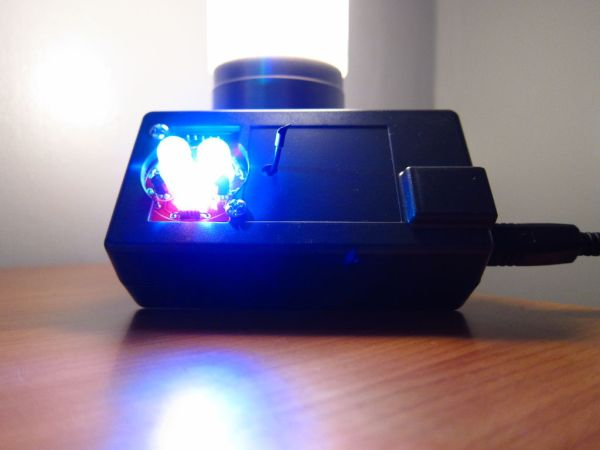# Sensing Elements for Current Measurements

Introduction

The fundamentals to translating the analog world into the digital domain reduces to a handful of basic parameters. Voltage, current, and frequency are electrical parameters that describe most of the analog world.  Current measurements are used to monitor many different parameters, with one of them being power to a load.

There are many choices of sensing elements to measure current to a load. The choices of current sensing elements can be sorted by applications as well as the magnitude of the current measured. This write up is part one of a three part series that discusses different types of current sensing elements. The focus of this paper is evaluating current measurements using a shunt (sense) resistor. The paper explains how to choose a sense resistor, discuss the inaccuracies associated with the sensing element and the paper discusses extraneous parameters that compromises the overall measurement.Element 1: Shunt Resistor

Shunt resistors are the most versatile and cost effective means to measure current. A shunt resistor cost ranges from a few pennies to several dollars. A shunt resistor’s price is differentiated by value, temperature coefficient, power rating and size. Shunt resistors commonly increase in cost for lower temperature coefficient (TC) and for higher power rating while offering precision features in a small package size.

With the knowledge of Ohm’s Law and the magnitude of the current to be measured, a shunt resistor is able to be designed into many applications. The simplicity of the design in process makes a resistor a versatile current sensing element.

In choosing a sense resistor value, the full scale voltage drop across the sense resistor and the maximum expected current measured for the application has to be known.

READ  Print Your Own Circuit Boards and Reflow SMD Components with the Voltera V-One

When possible, the voltage across the sense resistor should be kept to a minimum to lower the power dissipated by the sensing element. Low power dissipated by the sense resistor limits the heating of the resistor. A small temperature change to the sensor resistor results in a smaller resistance change versus all current sensing values.  The stability and accuracy of the sense resistor versus all currents improves with a constant value shunt resistor.

For more detail: Sensing Elements for Current Measurements